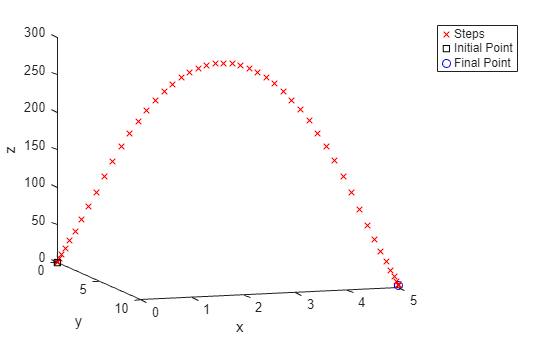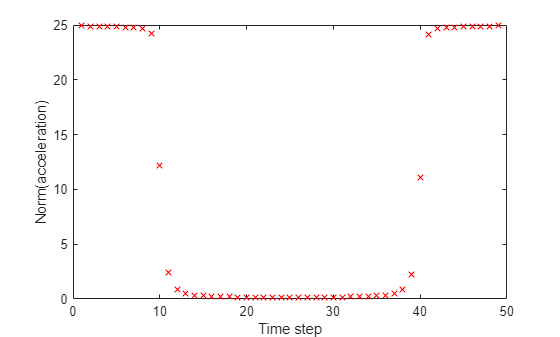# Discretized Optimal Trajectory, Problem-Based

This example shows how to solve a discretized optimal trajectory problem using the problem-based approach. The trajectory has constant gravity, limits on the applied force, and no air resistance. The solution process is to solve for the optimal trajectory over a fixed time $T$, and use that solution to find the optimal $T$, meaning the time that minimizes the cost. The final section shows how to include air resistance.

Compared to a nondiscretized optimization, such as the example Fit ODE, Problem-Based, the discretized version is not as accurate at solving an ordinary differential equation (ODE). However, the discretized version is not affected by noise in the variable-step ODE solver, as described in Optimizing a Simulation or Ordinary Differential Equation. The discretized version can also be easier to customize, and is straightforward to model. Finally, the discretized version can take advantage of automatic differentiation in the optimization process; see Effect of Automatic Differentiation in Problem-Based Optimization.

### Problem Description

The problem is to move an object from position ${p}_{0}$ at time $0$ to position ${p}_{F}$ at time $T$ using a controlled jet. Represent position as a vector $p\left(t\right)$, velocity as a vector $v\left(t\right)$, and applied acceleration as a vector $a\left(t\right)$. In continuous time, the equations of motion, including the force of gravity, are

`$\frac{dp}{dt}=v\left(t\right)$`

$\frac{dv}{dt}=a\left(t\right)+g*\left[0,0,-1\right]$.

Solve the problem by discretizing time. Divide time into $N$ equal intervals of size $t=T/N$. The position at time step $i$ is a vector $p\left(i\right)$, the velocity is a vector $v\left(i\right)$, and the applied acceleration is a vector $a\left(i\right)$. You can make a set of equations that represent an ODE model fairly accurately. Some approximate equations of motion are:

`$v\left(i\right)=v\left(i-1\right)+t\left(a\left(i-1\right)+g\right)$`

`$\begin{array}{l}p\left(i\right)=p\left(i-1\right)+t\left(\frac{v\left(i-1\right)+v\left(i\right)}{2}\right)\\ \phantom{\rule{1em}{0ex}}\phantom{\rule{0.5em}{0ex}}\phantom{\rule{0.2777777777777778em}{0ex}}=p\left(i-1\right)+tv\left(i-1\right)+{t}^{2}\frac{a\left(i-1\right)+g}{2}.\end{array}$`

The preceding equations integrate velocity using a two-point (trapezoidal rule) approximation, and integrate acceleration using a one-point (Euler) approximation. This integration scheme gives simple equations: the position and velocity at step $i$ depend only on the position, velocity, and acceleration at step $i-1$. The equations are also easy to modify for air resistance.

The boundary conditions are $p\left(1\right)={p}_{0}$, $p\left(N\right)={p}_{F}$, and $v\left(1\right)=v\left(N\right)=\left[0,0,0\right]$. Set the initial and final positions.

```p0 = [0 0 0]; pF = [5 10 3];```

The cost of using the jet to create force $a$ for time $t$ is $‖a‖t$. The total cost is the sum of the norms of the accelerations times $t$:

`$Cost=\sum _{i=1}^{N-1}‖a\left(i\right)‖t.$`

To convert this cost to a linear cost in optimization variables, create variables $s\left(i\right)$ and create associated second-order cone constraints:

`$\begin{array}{l}Cost=\sum _{i=1}^{N-1}s\left(i\right)t\\ ‖a\left(i\right)‖\le s\left(i\right).\end{array}$`

Impose additional constraints that the norm of the acceleration is bounded by a constant `Amax` for all time steps:

`$‖a\left(i\right)‖\le Amax.$`

These constraints are also second-order cone constraints. Because the constraints are linear or second-order cone constraints and the objective function is linear, `solve` calls the `coneprog` solver to solve the problem.

The following code creates an optimization problem for a fixed time T. The code incorporates the equations of motion as problem constraints. You can access the `setupproblem.m` function file by running this example using the live script. The function includes an air resistance argument; set `air = true` for a model with air resistance. For the definition of air resistance, see the section Include Air Resistance.

`type setupproblem`
```function trajectoryprob = setupproblem(T,air) if nargin == 1 air = false; end N = 50; g = [0 0 -9.81]; p0 = [0 0 0]; pF = [5 10 3]; Amax = 25; t = T/N; p = optimvar("p",N,3); v = optimvar("v",N,3); a = optimvar("a",N-1,3,"LowerBound",-Amax,"UpperBound",Amax); trajectoryprob = optimproblem; s = optimvar("s",N-1,"LowerBound",0,"UpperBound",3*Amax); trajectoryprob.Objective = sum(s)*t; scons = optimconstr(N-1); for i = 1:(N-1) scons(i) = norm(a(i,:)) <= s(i); end acons = optimconstr(N-1); for i = 1:(N-1) acons(i) = norm(a(i,:)) <= Amax; end vcons = optimconstr(N+1,3); vcons(1,:) = v(1,:) == [0 0 0]; if air vcons(2:N,:) = v(2:N,:) == v(1:(N-1),:)*exp(-t) + t*(a(1:(N-1),:) + repmat(g,N-1,1)); else vcons(2:N,:) = v(2:N,:) == v(1:(N-1),:) + t*(a(1:(N-1),:) + repmat(g,N-1,1)); end vcons(N+1,:) = v(N,:) == [0 0 0]; pcons = optimconstr(N+1,3); pcons(1,:) = p(1,:) == p0; if air pcons(2:N,:) = p(2:N,:) == p(1:(N-1),:) + t*(1+exp(-t))/2*v(1:(N-1),:) + t^2/2*(a(1:(N-1),:) + repmat(g,N-1,1)); else pcons(2:N,:) = p(2:N,:) == p(1:(N-1),:) + t*v(1:(N-1),:) + t^2/2*(a(1:(N-1),:) + repmat(g,N-1,1)); end pcons((N+1),:) = p(N,:) == pF; trajectoryprob.Constraints.acons = acons; trajectoryprob.Constraints.scons = scons; trajectoryprob.Constraints.vcons = vcons; trajectoryprob.Constraints.pcons = pcons; end ```

### Solve Problem for T = 20

Create and solve a trajectory problem for time $T=20$.

```trajprob = setupproblem(20); [sol,fval,eflag,output] = solve(trajprob)```
```Solving problem using coneprog. Optimal solution found. ```
```sol = struct with fields: a: [49x3 double] p: [50x3 double] s: [49x1 double] v: [50x3 double] ```
```fval = 192.2989 ```
```eflag = OptimalSolution ```
```output = struct with fields: iterations: 8 primalfeasibility: 3.2932e-07 dualfeasibility: 2.9508e-07 dualitygap: 1.7343e-08 algorithm: 'interior-point' linearsolver: 'prodchol' message: 'Optimal solution found.' solver: 'coneprog' ```

Plot the trajectory and norm of the acceleration by calling the `plottrajandaccel` helper function shown at the end of this example.

`plottrajandaccel(sol,p0,pF)`The acceleration is near that of gravity (9.81) for all times except those near the end, when the acceleration dips a bit.

### Find Minimal Cost

What time $T$ causes the cost to be minimal? For too small a time, such as $T=1$, the problem is infeasible, meaning it has no solution.

```myprob = setupproblem(1); [solm,fvalm,eflagm,outputm] = solve(myprob);```
```Solving problem using coneprog. Problem is infeasible. ```

Time 1.5 gives a feasible problem.

```myprob = setupproblem(1.5); [solm,fvalm,eflagm,outputm] = solve(myprob);```
```Solving problem using coneprog. Optimal solution found. ```

The `tomin` helper function at the end of this example sets up a problem for time `T` and then calls `solve` to calculate the cost of the solution. Call `fminbnd` on `tomin` to find the optimal time (lowest cost possible) in the interval $1.5\le T\le 10$.

`[Tmin,Fmin] = fminbnd(@(T)tomin(T,false),1.5,10)`
```Tmin = 1.9549 ```
```Fmin = 24.6102 ```

Obtain the trajectory for the optimal time `Tmin`.

```minprob = setupproblem(Tmin); sol = solve(minprob);```
```Solving problem using coneprog. Optimal solution found. ```

Plot the minimizing trajectory and acceleration.

`plottrajandaccel(sol,p0,pF)`The minimizing solution is nearly a "bang-bang" solution: the acceleration is either maximal or zero for all but two values.

### Plot Nonminimizing Trajectories

Plot the trajectories for a variety of times.

```figure hold on options = optimoptions("coneprog","Display","none"); for i = 1:10 T = 2*i; prob = setupproblem(T); sol = solve(prob,"Options",options); psol = sol.p; plot3(psol(:,1),psol(:,2),psol(:,3),'rx',"Color",[256-25*i 20*i 25*i]/256) end view([18 -10]) xlabel("x") ylabel("y") zlabel("z") legend("T = 2","T = 4","T = 6","T = 8","T = 10","T = 12","T = 14","T = 16","T = 18","T = 20") hold off```The shortest time (2) has a nearly direct trajectory on this scale. The intermediate times have large variations from a direct path. The largest time (20) also has a nearly direct path.

### Include Air Resistance

Change the model dynamics to include air resistance. Linear air resistance changes the velocity by a factor $\mathrm{exp}\left(-t\right)$ after time $t$. The equations of motion become

`$v\left(i\right)=v\left(i-1\right)\mathrm{exp}\left(-t\right)+t\left(a\left(i-1\right)+g\right)$`

`$\begin{array}{l}p\left(i\right)=p\left(i-1\right)+t\left(\frac{v\left(i-1\right)+v\left(i\right)}{2}\right)\\ \phantom{\rule{1em}{0ex}}\phantom{\rule{1em}{0ex}}=p\left(i-1\right)+t\left(\frac{1+\mathrm{exp}\left(-t\right)}{2}\right)v\left(i-1\right)+{t}^{2}\frac{a\left(i-1\right)+g}{2}.\end{array}$`

The problem formulation in the `setupproblem(T,air)` function for `air = true` has factors of `exp(-t)` in both the line defining the velocity constraint and the line defining the position constraint:

```vcons(2:N,:) = v(2:N,:) == v(1:(N-1),:)*exp(-t) + t*(a(1:(N-1),:) + repmat(g,N-1,1)); pcons(2:N,:) = p(2:N,:) == p(1:(N-1),:) + t*(1+exp(-t))/2*v(1:(N-1),:) + t^2/2*(a(1:(N-1),:) + repmat(g,N-1,1)); ```

Find the optimal time for the problem with air resistance.

`[Tmin2,Fmin2] = fminbnd(@(T)tomin(T,true),1.5,10)`
```Tmin2 = 1.9394 ```
```Fmin2 = 28.7967 ```

The optimal time is only slightly lower than the time in the problem without air resistance (1.94 instead of 1.95), but the cost `Fmin` is about 17% higher (28.8 instead of 24.6).

`compartable = table([Tmin;Tmin2],[Fmin;Fmin2],'VariableNames',["Time" "Cost"],'RowNames',["Original" "Air Resistance"])`
```compartable=2×2 table Time Cost ______ ______ Original 1.9549 24.61 Air Resistance 1.9394 28.797 ```

Plot the trajectory and acceleration for the optimal solution with air resistance.

```minprob = setupproblem(Tmin2,true); sol = solve(minprob);```
```Solving problem using coneprog. Optimal solution found. ```
`plottrajandaccel(sol,p0,pF)`With air resistance, the time of zero acceleration shifts to a later time step and is shorter. However, the solution is still nearly "bang-bang."

### Helper Functions

This code creates the `plottrajandaccel` helper function.

```function plottrajandaccel(sol,p0,pF) figure psol = sol.p; plot3(psol(:,1),psol(:,2),psol(:,3),'rx') hold on plot3(p0(1),p0(2),p0(3),'ks') plot3(pF(1),pF(2),pF(3),'bo') hold off view([18 -10]) xlabel("x") ylabel("y") zlabel("z") legend("Steps","Initial Point","Final Point") figure asolm = sol.a; nasolm = sqrt(sum(asolm.^2,2)); plot(nasolm,"rx") xlabel("Time step") ylabel("Norm(acceleration)") end```

This code creates the `tomin` helper function.

```function F = tomin(T,air) if nargin == 1 air = false; end problem = setupproblem(T,air); opts = optimoptions("coneprog","Display","none"); [~,F] = solve(problem,"Options",opts); end```